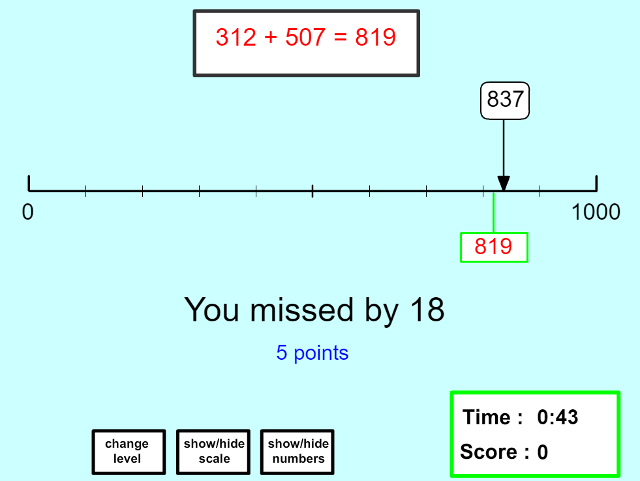## Placing Calculations on a Number Line

Place the answer to calculations on a number line. An enormous array of choice. Addition, subtraction, multiplication and division. Ranging from adding numbers to 10 to multiplying 4 digit numbers. Great for combining teaching calculations and approximations with developing an understanding of place value.